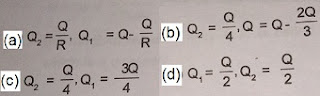## Services

Showing posts with label Electrostatics. Show all posts
Showing posts with label Electrostatics. Show all posts## Electrostatics : India Study Solution Test Series, solved Physics objective questions for JEE, NEET, other admission tests

### Physics - Electrostatics

India Study Solution Physics Test Series (Confidence Booster)
NEET, JEE, IIT, AIIMS, NTSE | Engineering, BDS, MBBS Admission Test
Hi Guys,
Welcome back to your own site India Study Solution
From today we are starting a new chapter Electrostatics from Physics which will have 30 sets of test series, each set containing 10 most important MCQ questions with their hints & solutions (given at the end).
(Please scroll down to find Electrostatics syllabus for NEET and JEE).
This is a very important chapter and so Friends, we would ask you to please bookmark this site or if you have not subscribed us yet then please subscribe before you forget (it is free) so that you get information whenever we publish more questions on this and other chapter / subject.
Hope it would help you to learn and enhance your confidence while preparing for NEET, JEE (IIT), AIIMS, COMEDK, NEST or any other Medical and Engineering Entrance Exams.
So, friends without wasting time let us start -

Electrostatics
India Study Solution Test Series – Set 1 (Q. No 1-10)
Question 1: A total charge Q is broken in two parts Q1 and Q2 and they are placed at a distance R from each other. The maximum force of repulsion between them will occur, when

Question 2: When the separation between two charges is increased, the electric potential energy of the charges
a. increases
b. decreases
c. remains the same
d. may increase or decrease

Question 3: Three charges +4q, -q and +4q are kept on a straight line at position (0, 0, 0), (a, 0, 0) and (2a, 0, 0) respectively. Considering that they are free to move along the x-axis only
a. all the charges are in stable equilibrium
b. all the charges are in unstable equilibrium
c. only the middle charge is in stable equilibrium
d. only the middle charge is in unstable equilibrium

Question 4: A soap bubble is given a negative charge, then its radius:
a. decreases
b. increases
c. remains unchanged
d. nothing can predicted as information is insufficient

Question 5: When a soap bubble is charged it
a. Bursts
b. Does not undergo any charge
c. Expands
d. Contracts

Question 6: Due to the motion of a charge, its magnitude
a. changes
b. does not change
c. increase (or) decreases depends on its speed
d. cannot be predicted

Question 7: Charges +q and –q are placed at points A and B respectively which are a distance 2 L apart, C is the midpoint between A and B. The work done in moving a charge +Q along the semicircle CRD is:

Question 8: Static electricity is produced by
a. Friction only
b. Induction only
c. Friction and induction both
d. Chemical reaction only

Question 9: A simple pendulum of period T has a metal bob which is negatively charged. If it is allowed to oscillate above a positively charged metal plate, its period will be
a. Remains equal to T
b. Less than T
c. Greater than T
d. Infinite

Question 10: If a positive charge is shifted from a low-potential region to a high –potential region, the electric potential energy
a. increases
b. decreases
c. remains the same
d. may increase or decrease

Electrostatics (Syllabus for NEET and JEE): Introduction, Electric Charges and their properties, Frictional Electricity, Charges and their Conservation, Unit of Charge, Conductors and Insulators, Presence of free Charges and bound Charges inside a Conductor, Charging by Induction, Coulomb’s Law, Dielectric Constant,  Forces between Multiple Charges, Electric Field, Electric Field lines, Electric Flux, Electric Dipole, Dipole in a Uniform External Field, Torque on a Dipole in a uniform electric field,  Continuous Charges distribution, Gauss’s Law and its applications, Electrostatic Potential, Potential due to Point Charge / Electric dipole / System of charges, Equipotential Surfaces, Potential Energy of a System of Charges/ External Field,
India Study Solution Test Series
electrostatics
Hints and Solutions of Physics Electrostatics MCQ Test Series – Set 1 (Q. No.1–10)

Answer 4: b (Hint: On the surface of bubble same charge will distribute, so there is repulsion of charges so radius of bubble increases).